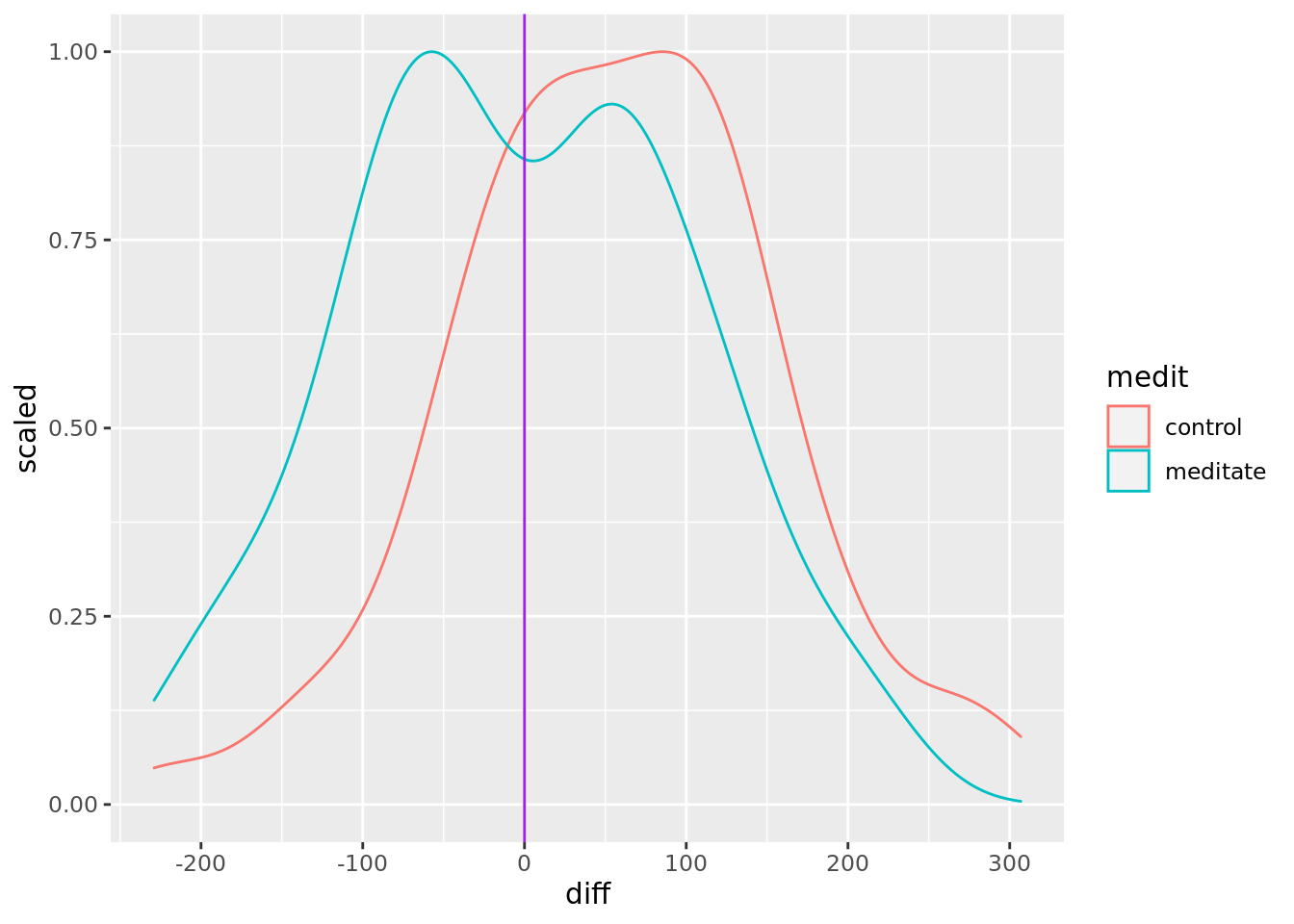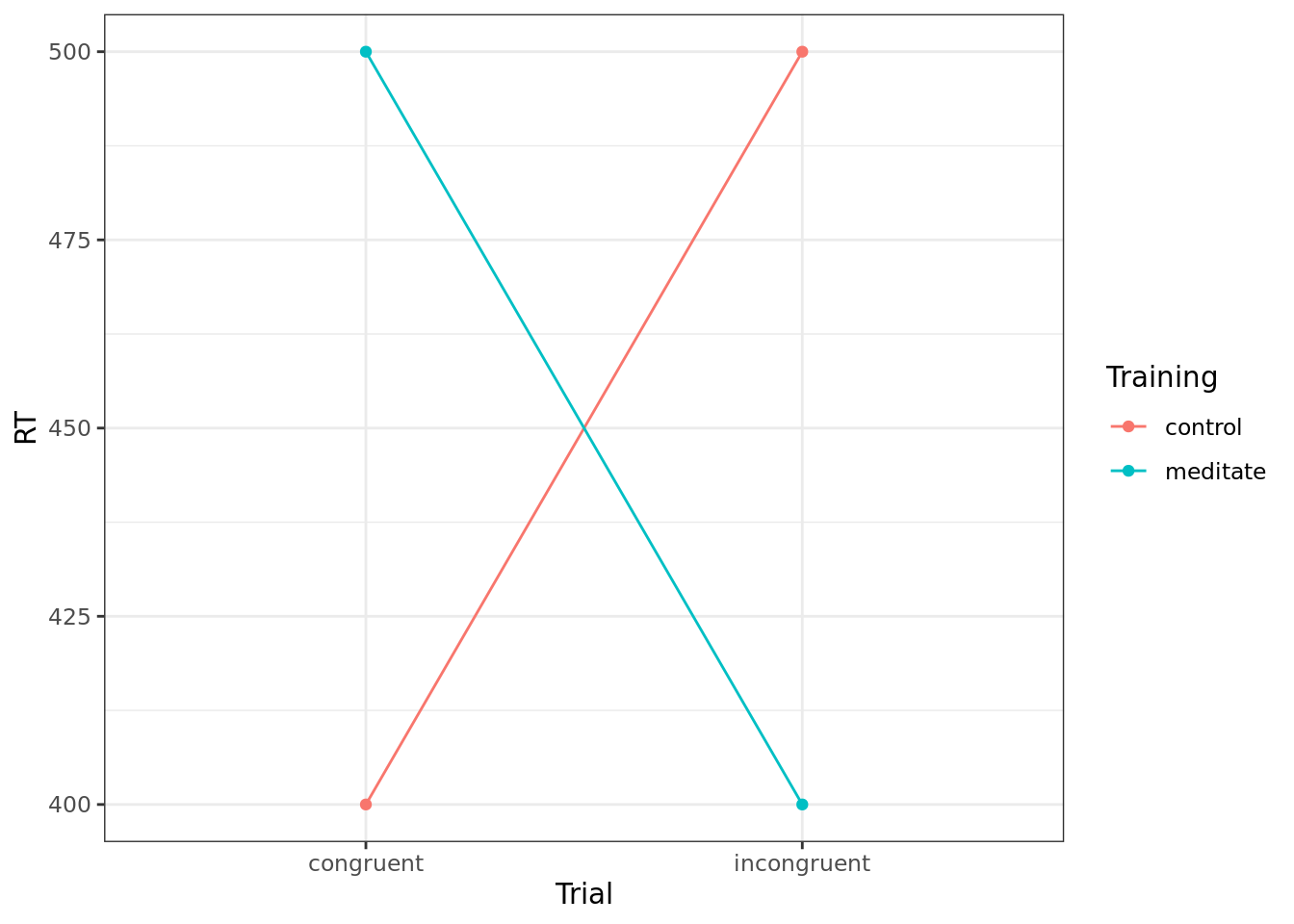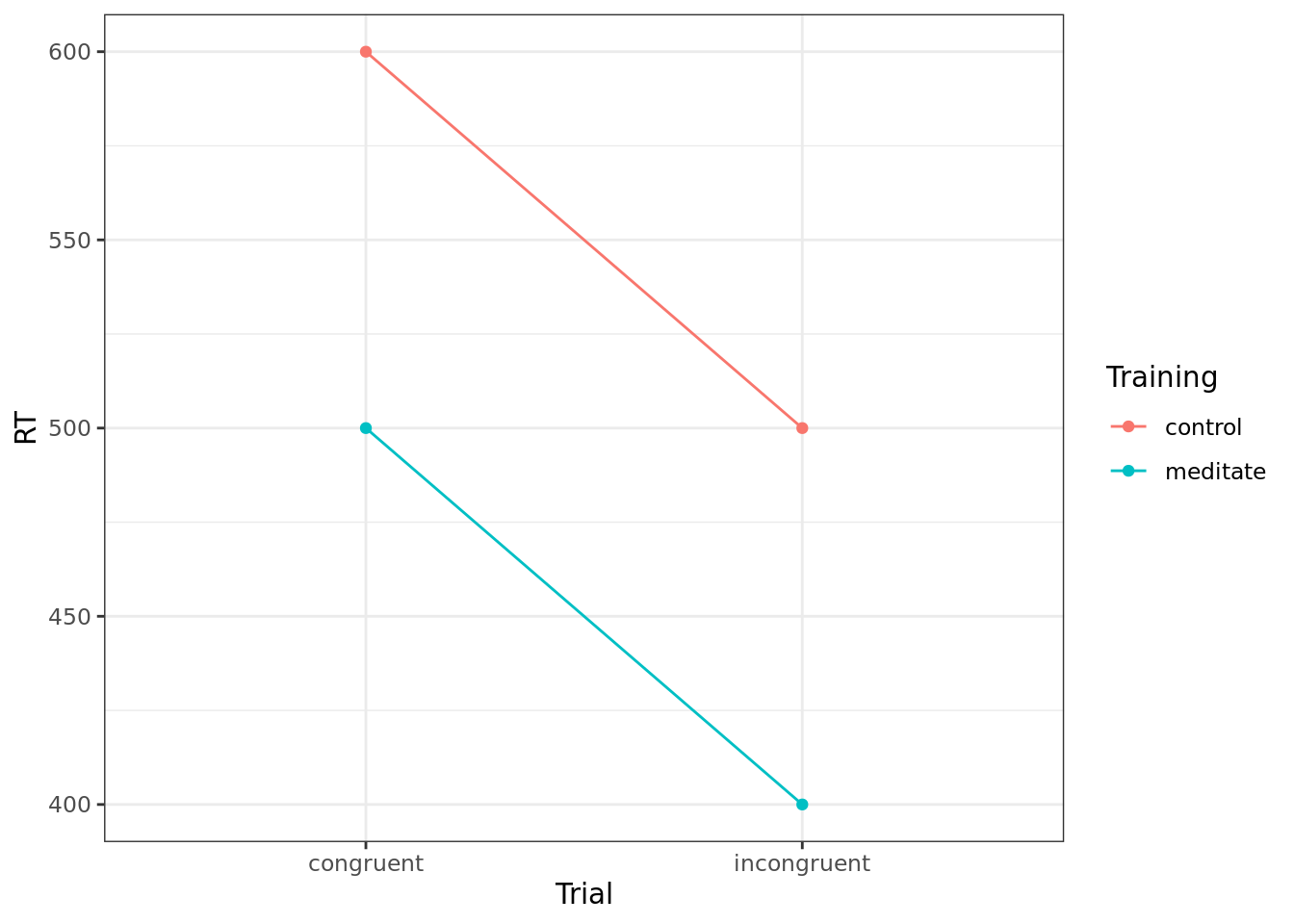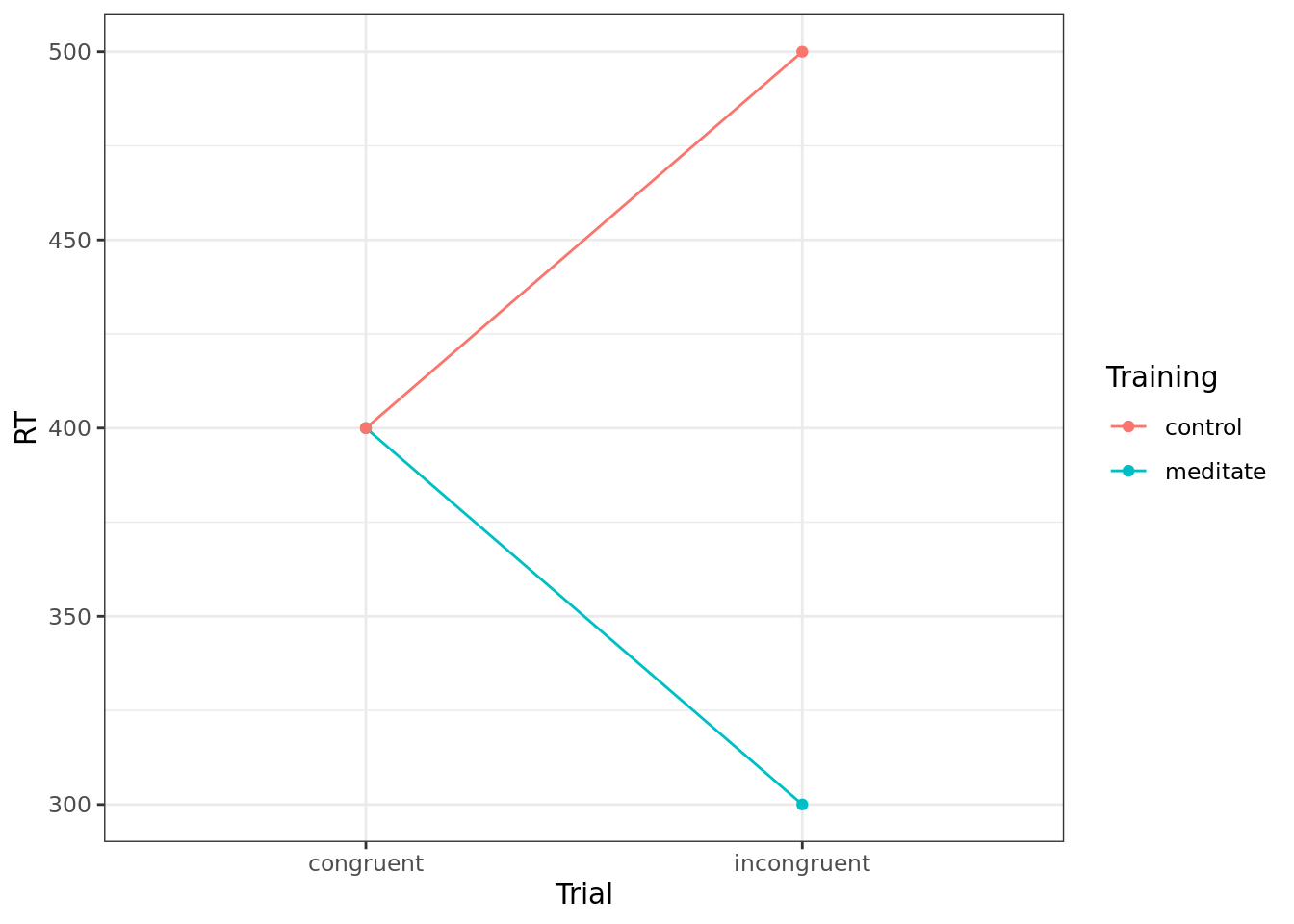# Introduction

In the within-subject differences worksheet, we used a Bayesian within-subjects ANOVA to test for an effect of word-picture congruence in word naming. Specifically, we looked at an experiment in which people had to read words out loud as quickly as possible. On some trials, those words were accompanied by congruent pictures (e.g. the word ‘dog’ and a picture of a dog). On other trials, the words were accompanied by an incongruent picture (e.g. the word ‘dog’ and a picture of a pencil). Participants were instructed to ignore the pictures … but we found they could not do so. Reaction times were longer for incongruent trials than congruent trials, and there was substantial Bayesian evidence for that difference. In fact the Bayes Factor was over 40 million ($$BF = 4.4 \times 10^{7}$$).

These kinds of congruency effects are well known in psychology, and demonstrating them once again was not the main point of this experiment. Instead, the researchers were interested in the idea that learning how to meditate might increase your ability to attend to just the relevant aspects of a task. If this were true, the congruency effect would be smaller for those trained in meditation than for those who did not receive such training. In other words, the difference between the incongruent reaction time and the congruent reaction time would be smaller for meditators than non-meditators.

To test this idea, the experimenters randomly allocated 140 people to a week-long meditation training course, while another 140 people were randomly allocated to a no-training control condition. All these people then did a word-naming task. In this worksheet, and the next one, we’ll look at how to analyse data from this kind of experiment.

Specifically, our goal in these two worksheets is to work out the level of evidence for the hypothesis that meditation training reduces the size of the congruency effect. In order to do this, we need to make use of the concept of an interaction. This is a concept that for some people can take a while to grasp, so we’re going to take it in little steps, and approach it in a few different ways. By the end of these two worksheets, things should be clearer.

# Getting started

This worksheet uses the same git repository as the preprocessing and within-subject differences worksheets. Go back to your R project that contains this git repository now - you named it rminr-data.

Open a new blank script, and save it as anova2.R. Enter all your commands into this script and run them from there. Save the script regularly.

The first step is to make sure you have loaded the data, and have loaded the R packages you need.

library(tidyverse)
library(BayesFactor)
words <- read_csv("wordnaming2.csv")

Take a look back at the description of this data frame to remind yourself what it contains.

# Preprocessing

As before, we need to preprocess this large data set before we can analyse it.

## Filtering

In the full experiment, there were actually three between-subject conditions: meditation training, no training (“control”), and relaxation training. In this first example, we’re only going to look at the first two of those conditions: meditate and control. So,

WRITE A COMMAND that removes the participants in relax condition from the words data frame. It is the medit column of that data frame that contains the information about which condition each participant was in. Use the filter command to do this, and put the result into a new data frame called MCwords. As the filter command sets which data to keep, you may find the != operator useful here – it means ‘not equal to’. If you need to revise how to do this, take a look back at the preprocessing worksheet.

EXPECTED OUTPUT: A new data frame called MCwords will be in your Environment. It will have 25,200 rows and 10 columns.

The full experiment also included three types of trial: congruent, incongruent, and neutral. The first two are described above. In neutral trials, the word is presented alone, without a picture. In this first example, we’re only going to look at the congruent and incongruent trials. So,

WRITE A COMMAND that removes the neutral trials from the MCwords data frame, and puts the filtered data into a new data frame called MCwordsCI. It is the congru column that contains information about trial type.

EXPECTED OUTPUT: A new data frame called MCwordsCI will be in your Environment. It will have 16,800 rows and 10 columns.

## Summarising

The data frame MCwordsCI is still very long, because it contains every trial for every participant. What we want to know, for each participant, is their mean reaction time on congruent and incongruent trials (i.e. two numbers per person). So,

WRITE A COMMAND to group the data by participant ID (subj), trial type (congru) and between-subjects condition (i.e. meditation versus control, medit). Get the mean reaction times using the summarise command, and put this summarised data into a new data frame called MCwordsCIsum. If you need a reminder of how to group and summarise data, take a look at the preprocessing worksheet. The column containing the RT data in this new data frame will need to have the name rt, because we assume this later on. You can set the column name within the summarise command. For example, summarise(acc = mean(acc)) takes the mean of the column acc and puts the answer into a column called acc in your new data frame.

EXPECTED OUTPUT: A new data frame called MCwordsCIsum will be in your Environment. It will have 560 rows and 4 columns. There will be two rows for each participant, one for incongruent trials, one for congruent trials. Each participant will be identified as being in either the meditate or control condition.

## Pivoting and mutating

Exactly as we did in the within-subject differences worksheet, we can now use the pivot_wider and mutate commands to work out a difference score for each participant. This gives us the size of the congruency effect for that participant, i.e. how much more time they take on incongruent trials than congruent trials (on average).

Enter these commands into your R script and run them:

medidiff <- MCwordsCIsum %>%
pivot_wider(names_from = congru, values_from = rt) %>%
mutate(diff = incong - cong)

Take a look at the medidiff data frame in your Environment. You’ll see there is now one row for each participant, with a difference score in the diff column. You may also notice that, in the meditate condition, there are about an equal number of positive and negative differences. Things look different in the control condition, where positive scores are much more likely than negative scores. So, on a quick look, it seems like the congruency effect might be (on average) smaller for those with meditation training.

# Density plot of differences

We can look at this difference in congruency effect more closely using a density plot. We make such a plot in the same way as we have on many previous occasions.

Enter these commands into your R script and run them:

medidiff %>% ggplot(aes(diff, colour=medit)) +
geom_density(aes(y=..scaled..)) +
geom_vline(xintercept = 0, colour = 'purple')This plot is very much like the one we drew in the within-subject differences worksheet, and the curve for the control condition is identical. So, as before, we can see that in the control condition, most congruency scores are positive, although there is of course a range (from about -200 to +300 ms).

The new part of this graph is that we also have a curve for the meditation condition. It is the colour=medit part of the command that causes this to happen - it draws one curve for each level of the medit factor, and gives each a different colour.

We can see that the curve for the meditate condition is approximately centred on zero. In other words, on average, there is no congruency effect after meditation training.

# Understanding interactions

To summarise, we’ve found that the congruency effect is smaller after meditation training than after no training. The congruency effect is, as we have discussed, calculated as a difference between two average reaction times - the reaction time on incongruent trials minus the reaction time on congruent trials. This difference is smaller after meditation training than after no training. So, the results of this experiment can be described as a difference of differences. The RT difference is smaller for meditation than for control participants.

The phrase difference of differences is a bit clumsy, so we often use another, jargon, word for it. We say that the results of this experiment show an interaction between trial type (congruent, incongruent) and training type (meditation, control). This is just another way of saying the size of the difference between trial types is affected by training type. This is what our density plot of differences (above) shows.

## Factorial designs, main effects, and interactions

We normally talk about interactions in the context of experiments that have a factorial design. An experiment has a factorial design if more than one factor is manipulated, and all combinations of those factors are tested. For example, the meditation and attention experiment we’ve been looking at has a factorial design. It has two factors. The first factor is type of pre-training (meditation versus none). The second factor is type of test trial (congruent versus incongruent). The experiment has a factorial design because we have data for all four combinations of trial types (congruent, incongruent) and pre-training conditions (meditation, none).

With a two-factor experiment like this one, there are three basic analysis questions we can ask:

1. Averaging over different trial types (congruent, incongruent), does meditation affect reaction times? This is known as the main effect of meditation.

2. Averaging over different pre-training conditions (meditation, control), does trial type (congruent, incongruent) affect reaction times? This is known as the main effect of trial type (or the main effect of congruency, in this case).

3. Do these two main effects interact? So, for example, is the congruency effect smaller in the meditation condition than the control condition? This is known as the interaction.

For each of these questions, the answer can be either ‘yes’ or ‘no’. If we don’t have enough data, the answer could also be ‘we don’t know’, but we’ll come back to that later. So, when we analyse a two-factor experiment, there are eight different results we could get:

Main effect 1: (e.g. meditation) Main effect 2: (e.g. congruence) Interaction
no no no
no no yes
no yes no
no yes yes
yes no no
yes no yes
yes yes no
yes yes yes

## Exercise

Below, you will find three graphs. Each shows four mean reaction times - mean reaction time for incongruent and congruent trials for both the meditation condition and the control condition. For each graph, decide whether there is a main effect of meditation, whether there is a main effect of congruence, and whether there is an interaction. Write your answers into PsycEL in each case.

### Graph 1

We’ll work through the first example together:Is there a main effect of trial type (congruence)? No. There are two reaction times for the congruent trial type - 400ms (bottom left) and 500ms (top left). The average of these is 450 ms. Similarly, there are two reaction times for the incongruent trial type - 400 ms (bottom right) and 500 ms (top right). The average of these is also 450 ms. So, the average reaction time for congruent trials is the same as the average reaction time for incongruent trials, which means there is no main effect of trial type.

Is there a main effect of training type (meditation)? No. There are two reaction times for the control group - 400 ms (bottom left) and 500 ms (top right). The average is 450 ms. There are two reaction times for the meditate group - 500 ms (top left) and 400 ms (bottom right). The average is 450 ms. So, the average reaction time in the two groups is the same, so there is no main effect of training type.

Is there an interaction? Yes. Remember that an interaction is a difference of differences. In the control condition the incongruent RT is 500 ms and the congruent RT is 400 ms, so incongruent - congruent = 100 ms. In the meditate condition, the incongruent RT is 400 ms and the congruent RT is 500 ms, so incongruent - congruent = - 100 ms. These two differences are different (one is +100 ms, the other is -100 ms), so there is an interaction. Another way of easily spotting an interaction is if the lines on the graph are at different angles. In the graph above, the two lines have very different angles (one goes up from left to right, the other goes down). Unless the lines are parallel there is an interaction.

### Graph 2

Go through the same process to work out whether, in the graph below, there is a main effect of training, a main effect of trial type, and an interaction.### Graph 3

Now do the same for this graph. At first glance, it might seem like there are only three data points on this graph. The congruent RT for the meditate group is identical to the congruent RT for the control group, and so only one point is visible.### Line graphs

In the next part of this exercise, we’ll use R to generate another graph of main effects and interactions. We haven’t done line graphs before, so first let’s have a look at how the three graphs above were produced.

The data points for these graphs were loaded from a CSV file. There’s a copy of this file in the same git repository that we’ve being using throughout this worksheet, so the first thing to do is load it.

Enter this command into your R script and run it:

interact <- read_csv("interact.csv")

Take a look at this data frame by clicking on it in your environment. You’ll find it has the following columns:

Column Description Values
Graph ID number for the graph 1-4
Training training condition meditate, control
Trial trial type congruent, incongruent
RT reaction time a number

Graphs 1 to 3 are the three shown above. There are no reaction times for Graph 4, because you’ll be adding those later.

#### Reproducing Graph 1

First, we’re going to reproduce Graph 1. The first step is to filter the data so it just includes the first graph.

Enter this command into your R script and run it:

graph1 <- interact %>% filter(Graph == 1)

Once that’s done, we can make a line graph, like this.

Enter these commands into your R script and run them:

graph1 %>%
ggplot(aes(x = Trial, y = RT, group = Training)) +
geom_line(aes(colour=Training)) +
geom_point(aes(colour=Training)) +
theme_bw()